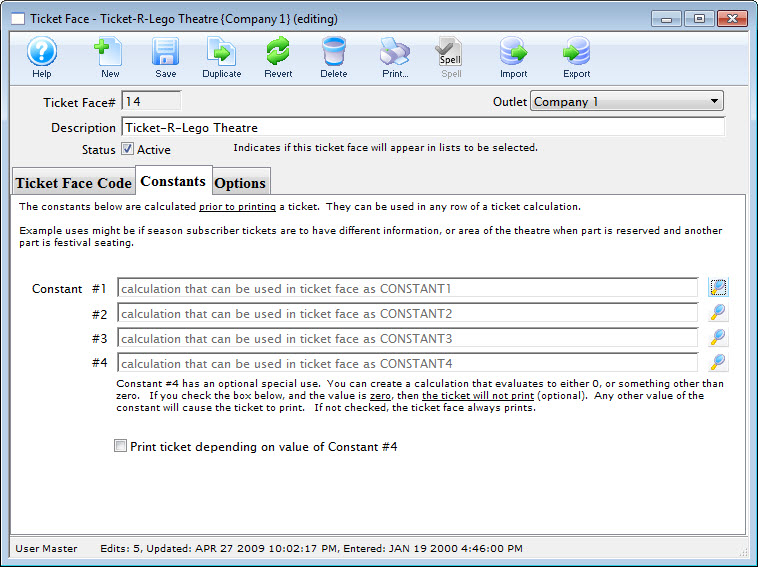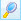# Constants Tab

You use constants to add to ticket face equations. Constants replace long lines of equation that need to be repeated or in conjunction with pick statements. A pick statement may read if the constant is not met to print one thing on the ticket but if the constant is met to print something completely different. Constants allow for more editing options and can be used as many times as is needed in the ticket face.## Parts of the Constants Tab

 Constant #1 First constant equation. Constant #2 Second constant equation. Constant #3 Third constant equation. Constant #4 Fourth constant equation.Opens the constant equation window. For more information on creating constants click here. Print Ticket Depending on Value of Constant #4 If the value of constant #4 equals zero the ticket face will not print.